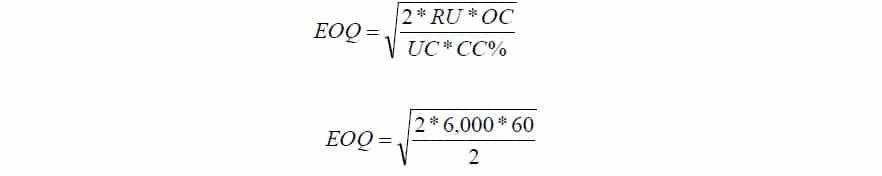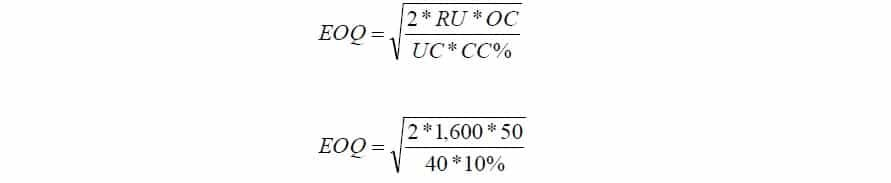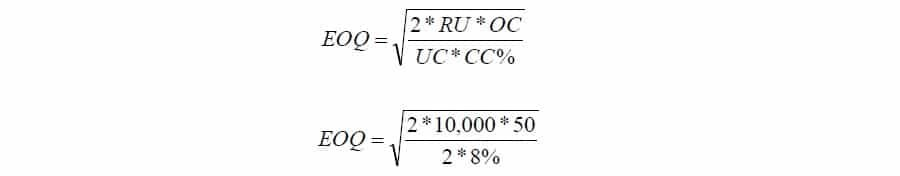Select Page

# Economic Order Quantity Problems and Solutions

## Problem # 1:

Calculate Economic Order Quantity (EOQ) from the following:

Annual consumption           6,000 units

Cost of ordering                 Rs. 60

Carrying costs                    Rs. 2

### Solution:EOQ =600 Units

>> More Reading Economic Order Quantity.

## Problem # 2:

From the following particulars, calculate the Economic Order Quantity (EOQ):

Annual requirements          1,600 units                          Cost of materials per units                      Rs. 40

Cost of placing and receiving one order:      Rs. 50       Annual carrying cost for inventory value       10%

### Solution:EOQ = 200 Units

>> More Reading Inventory Management.

## Problem # 3:

Calculate EOQ from the following?

Consumption during the year = 600 units              Ordering cost Rs. 12 per order

Carrying cost 20%                                                  Selling Price per unit Rs. 20

### Solution:Economic Order Quantity = 379 Units

>> Practice Inventory Management Problems and Solutions.

## Problem # 4:

A manufacturer buys certain equipment form suppliers at Rs. 30 per unit. Total annual needs are 800 units. The following further data are available:

Annual return on investments 10%                    Rent, insurance, storing per unit per year Rs. 2

Cost of placing an order Rs. 100

Required: EOQ

### Solution:EOQ = 200 Units

>> More Reading Cost of Goods Sold.

## Problem # 5:

From the figures given below, calculate Economic Order Quantity (EOQ) and Total cost at EOQ?

Total consumption of material per year         10,000 kgs                Buying cost per order Rs. 50

Unit cost of material                                        Rs. 2 per kg            Carrying and storage cost 8%

### Solution:EOQ = 2,500 Units

Total Inventory Cost = [Fixed ordering cost (F) * Number of Order per year N] + [Carrying Cost (C)* EOQ/2]

Total Inventory Cost = [50 * 10,000/2,500] + [(2*0.08)* 2,500/2]

Total Inventory Cost = 200 + 200

Total Inventory Cost = Rs.  400

>> More Reading Costing .

## Related Topics

Economic Order Quantity PDF

Economic Order Quantity

Economic Order Quantity MCQs

## Related Courses

Principles of Accounting

Cost Accounting

Principles of Finance

# 1 Comment

1.Excellent ѕite ｙou have here.. It’s diffіcult to find
gooԁ quality writing liҝe yours these days. I serioᥙsly apρreciate individuals like
you! Take care!!